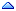Home » SQL & PL/SQL » SQL & PL/SQL » unix date to "oracle date"
unix date to "oracle date" Thu, 31 January 2002 07:51Waylan Messages: 1Registered: January 2002 Junior Member
Is there a way to turn a unix date thats in a table into oracle date in the sql. The calculation would be the oposite of (sysdate - to_date('01-01-1970','DD-MM-YYYY'))*60*60*24
Re: unix date to "oracle date" [message #18457 is a reply to message #18454] Thu, 31 January 2002 10:16andrew again Messages: 2577Registered: March 2000 Senior Member
From the NET somewhere...
```CREATE OR REPLACE FUNCTION Unix_To_Oracle_Date
(
p_unix_date IN NUMBER,  -- Range of >= 0
p_num_hr_gmt_diff IN PLS_INTEGER    -- Range of -24 to +24

) RETURN DATE AS

/*
Function Name:    Unix_To_Oracle_Date

Purpose:          To calculate an Oracle date based on a Unix GMT date
in seconds, since the epoch of Unix (January 1st, 1970).

The date returned will be based on the number of hours
the oracle database system clock is either ahead or behind
the Greenwich Mean Time line.

If the parameters do not fall within the specified ranges
then the returned date will be NULL.

Developer Name:   Giovanni Jaramillo
Developer Email:  Giovanni@CheckOut.com
Creation Date:    Copyright ? CheckOut.com December 2, 1999

Parameters:       p_unix_date of NUMBER type (Range is > 0 only)
p_num_hr_gmt_diff of PLS_INTEGER type (Range -24 to +24)

Input:            NONE
Output:           NONE
Returned value:   DATE := Unix_To_Oracle_Date(p_Unix_Date);

*/

-- CONSTANTS

-- These 2 CONSTANTS below are of POSITIVE type to indicate that they
-- CANNOT be less than 1

c_SECONDS_IN_DAY CONSTANT POSITIVE := 86400;
c_HOURS_IN_DAY CONSTANT POSITIVE := 24;

c_UNIX_EPOCH_DATE_TIME_STRING CONSTANT VARCHAR2(50) := '01/01/1970 00:00:00';
c_DATE_FORMAT CONSTANT VARCHAR2(50) := 'MM/DD/YYYY HH24:MI:SS';

-- VARIABLES

v_sql_code PLS_INTEGER;
v_sql_error_message VARCHAR2(512);

-- The 2 variables have to be of NUMBER type because a PLS_INTEGER divided by
-- another PLS_INTEGER can result in a fraction, thus the result must be of
-- NUMBER type only

v_unix_seconds NUMBER;
v_gmt_time_gap NUMBER;

v_return_value DATE;

BEGIN

IF(NOT (p_num_hr_gmt_diff < (-c_HOURS_IN_DAY)) AND
NOT (p_num_hr_gmt_diff > (c_HOURS_IN_DAY))) THEN

v_unix_seconds := (p_unix_date / c_SECONDS_IN_DAY);
v_gmt_time_gap := (p_num_hr_gmt_diff / c_HOURS_IN_DAY);

v_return_value := TO_DATE(c_UNIX_EPOCH_DATE_TIME_STRING, c_DATE_FORMAT) +
v_unix_seconds + v_gmt_time_gap;
ELSE
v_return_value := NULL;
END IF;

RETURN(v_return_value);

EXCEPTION

WHEN OTHERS THEN
v_sql_code := SQLCODE;
v_sql_error_message := SQLERRM(v_sql_code);

DBMS_OUTPUT.PUT_LINE('OTHER ERROR');
DBMS_OUTPUT.PUT_LINE(v_sql_error_message);

END Unix_To_Oracle_Date;
```
 Previous Topic: Using variables for accessing tables in pl/sql Next Topic: Problems when trying to use SYS_GUID()
Goto Forum:

Current Time: Thu Sep 21 10:15:17 CDT 2023